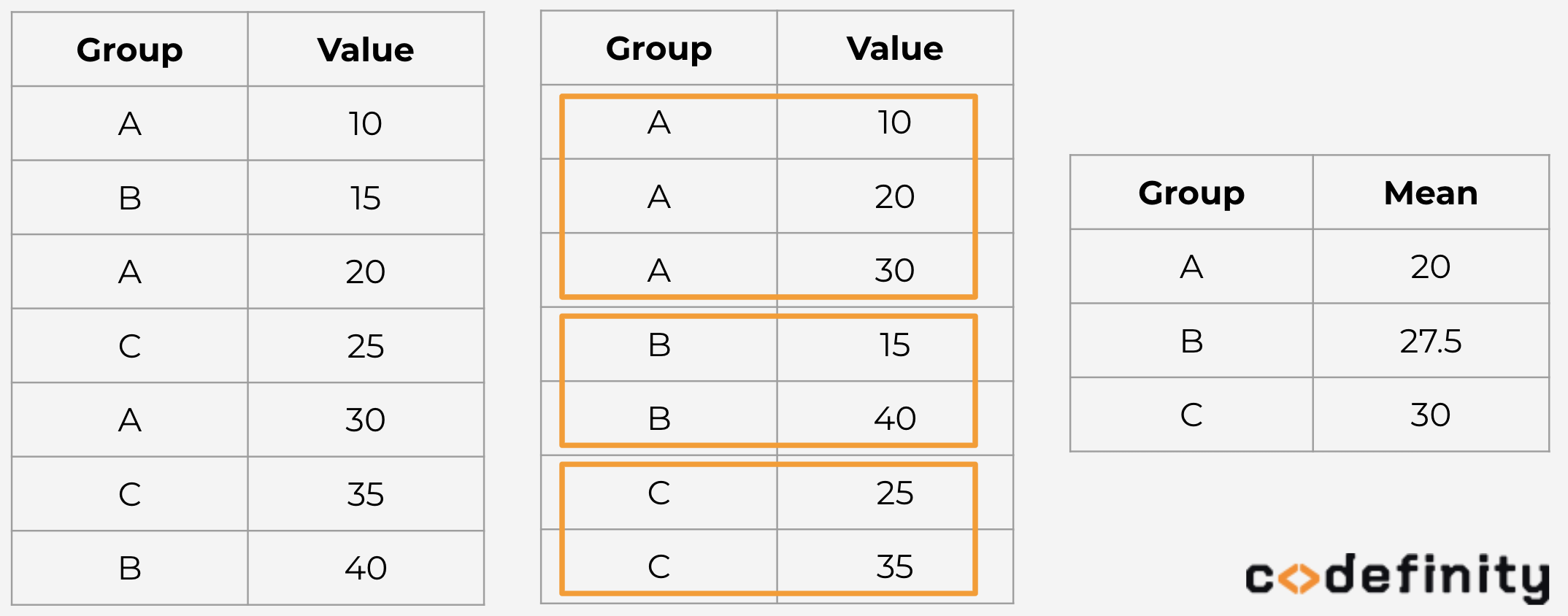Course Content

Data Manipulation using pandasWhat is Grouping Data?

When you read some articles with statistical information, you may often meet aggregated by groups data. For instance, average salary across each country/region.

By this time, you have learned how to aggregate entire data (like, `.mean()` or `.max()` methods). The result was always a single number - aggregated measurement for all the data. Grouping data will 'rearrange' values by unique records within specific column (group) and then perform respective calculations for values across each group. Look at the picture below to understand how can means across groups be calculated.As you can see, grouping gathers data by categories and then calculates mean value.

Section 3.

Chapter 1